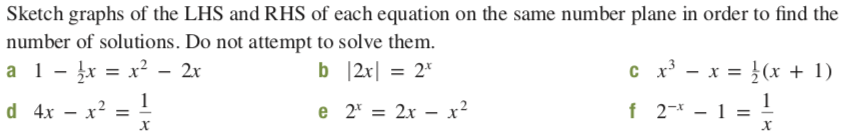# Sketch graphs of the LHS and RHS of each equation on the same number plane in order to find thenumber of solutions. Do not attempt to solve them.c x - x = }(x + 1)a 1- įx = x² - 2xb 2x| = 2*d 4x – x? = !- x²f 2-* - 1e 2* = 2x – x²

Question
4 views

b

solve this mathematically using high school knowledgehelp_outlineImage TranscriptioncloseSketch graphs of the LHS and RHS of each equation on the same number plane in order to find the number of solutions. Do not attempt to solve them. c x - x = }(x + 1) a 1- įx = x² - 2x b 2x| = 2* d 4x – x? = ! - x² f 2-* - 1 e 2* = 2x – x² fullscreen
check_circle

Step 1

### Want to see the full answer?

See Solution

#### Want to see this answer and more?

Solutions are written by subject experts who are available 24/7. Questions are typically answered within 1 hour.*

See Solution
*Response times may vary by subject and question.
Tagged in

### Math